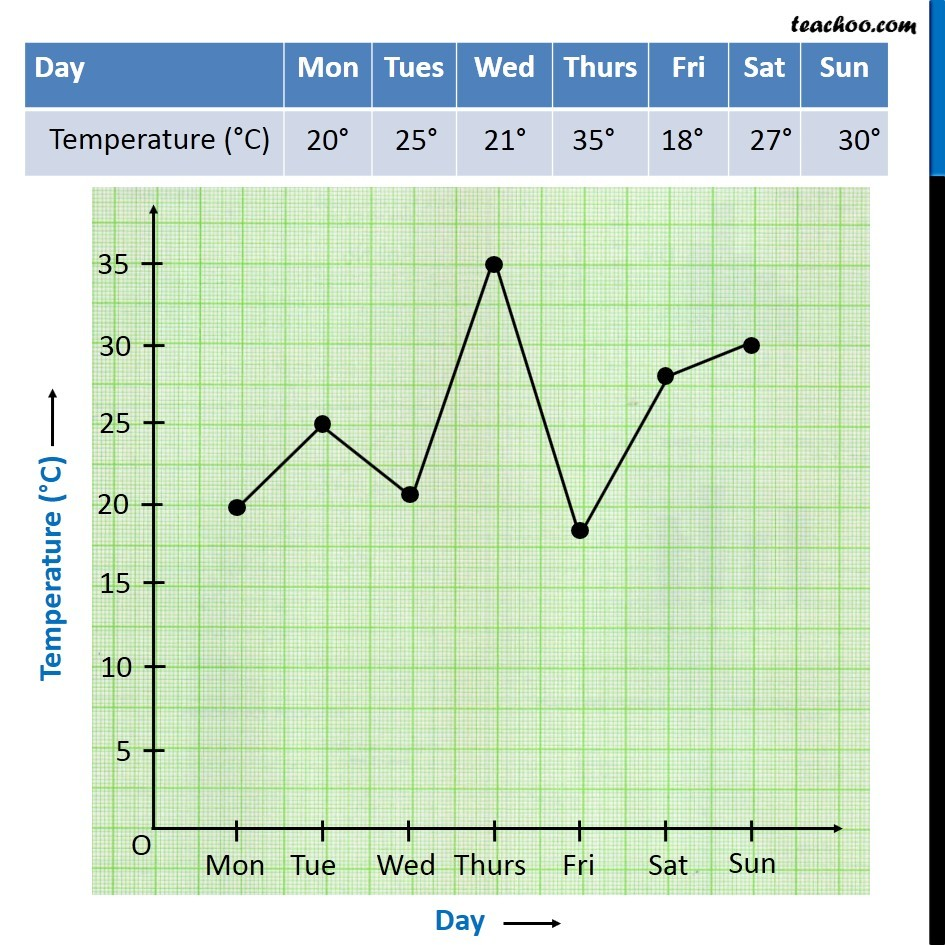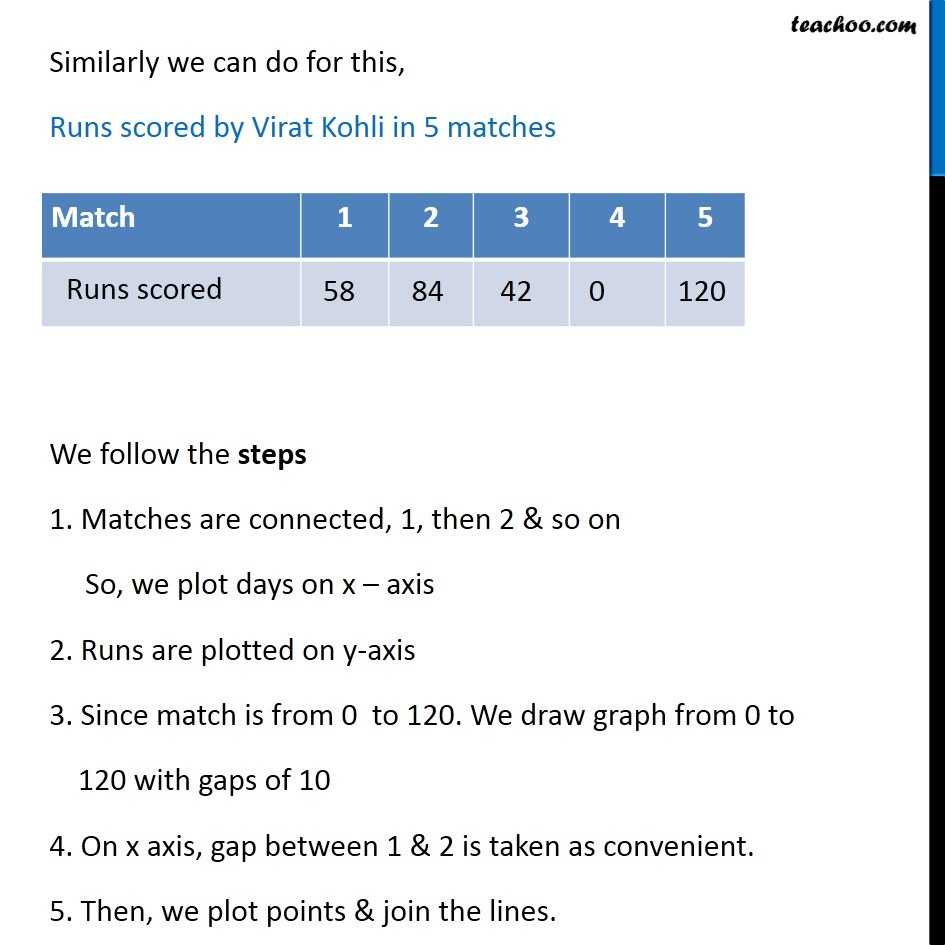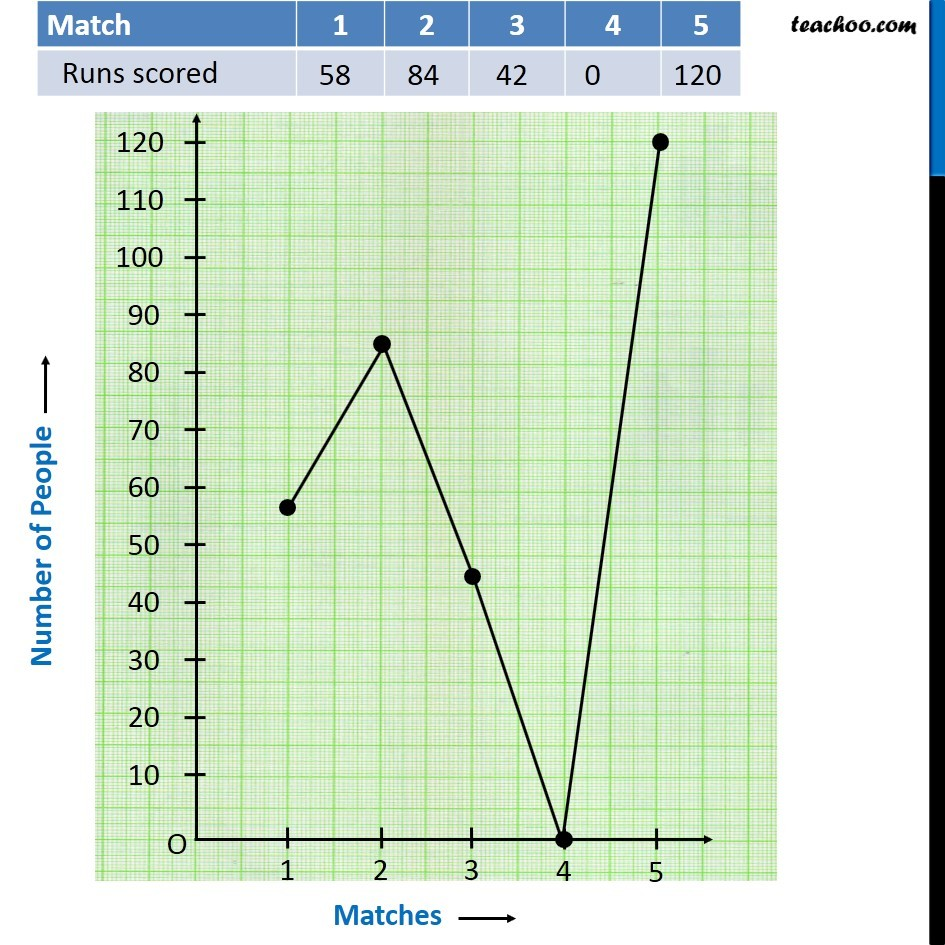Making Line Graph

Chapter 15 Class 8 Introduction to Graphs
Concept wise

How to make a line graph?

 Day Mon Tues Wed Thurs Fri Sat Sun Temperature (°C) 20° 25° 21° 35° 18° 27° 30°

1. Days are connected, first Monday, then Tuesday & so on So, we plot days on x – axis
2. Temperature is plotted on y-axis
3. Since temperature is from 18° to 35°.We draw graph from 0 to 35 with gaps of 5
4. On x axis, gap between Monday & Tuesday is taken as convenient.
5. Then, we plot points & join the lines.Get live Maths 1-on-1 Classs - Class 6 to 12

### Transcript

How to make line graph?Suppose we are given an Example 6 We follow the steps 1. Days are connected, first Monday, then Tuesday & so on So, we plot days on x – axis 2. Temperature is plotted on y-axis 3. Since temperature is from 18° to 35°. We draw graph from 0 to 35 with gaps of 5 4. On x axis, gap between Monday & Tuesday is taken as convenient. 5. Then, we plot points & join the lines. Similarly we can do for this, Runs scored by Virat Kohli in 5 matches We follow the steps 1. Matches are connected, 1, then 2 & so on So, we plot days on x – axis 2. Runs are plotted on y-axis 3. Since match is from 0 to 120. We draw graph from 0 to 120 with gaps of 10 4. On x axis, gap between 1 & 2 is taken as convenient. 5. Then, we plot points & join the lines.